# Shear Stress

## Shear Stress

Short-range forces, such as viscous forces, have a molecular origin and are, as a result, generally negligible unless there is physical contact between parts of the fluid. They can be approximated by forces on the surface of each part of the fluid and lead to the concept of stress in a fluid. If a force F acts on a surface S of a fluid with unit outer normal n (so n is the vector of unit magnitude which is normal to S and oriented outwards from the fluid) then, if S is small enough:
(1)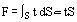where t denotes the stress vector. Note that t acts at a point whereas F does not. Because, however, t varies with the orientation of S, that is with n, it is not a property at a point. Thus it is appropriate [see Richardson (1989)] to introduce the stress tensorτ such that:
(2)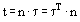The stress tensor τ at a point is independent of the orientation of any surface through the point: it is, therefore, a property at a point. The sign convention for stress is:
• t · n = (n · t) · n > 0 corresponds to tension;
• t · n = (n · t) · n < 0 corresponds to compression.
In a rectangular or Cartesian coordinate system (x,y,z):
(3)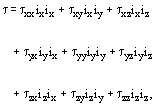where ix, iy and iz denote unit vectors in the x-direction, y-direction and z-direction, respectively (see Figure 1). The components of τ may be interpreted thus:
• τxx is the x-component of force per unit area exerted across a plane surface element normal to the x-direction;
• τxy is the y-component of force per unit area exerted across a plane surface element normal to the x-direction; and so on.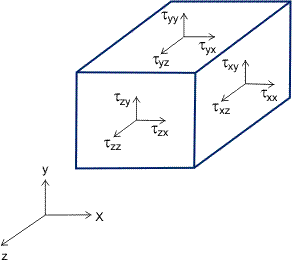Figure 1. Stress components.
(Precisely analogous interpretations can be given to components of stress in any orthogonal coordinate system.) Each component with identical suffices (τxx, τyy and τzz) is called a normal stress (qv) since it represents a force in a direction per unit area normal to that direction. Each other component with different suffices (τxy, τyx, τxz, τzx, τyz, and τzy) is called a shear stress.
For nonpolar fluids, that is fluids for which the angular momentum is just the moment of the linear momentum, it can be shown [see Richardson (1989)] that angular momentum conservation implies that:
(4)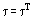It then follows that τxy = τyx, τxz = τzx, and τyz = τzy.